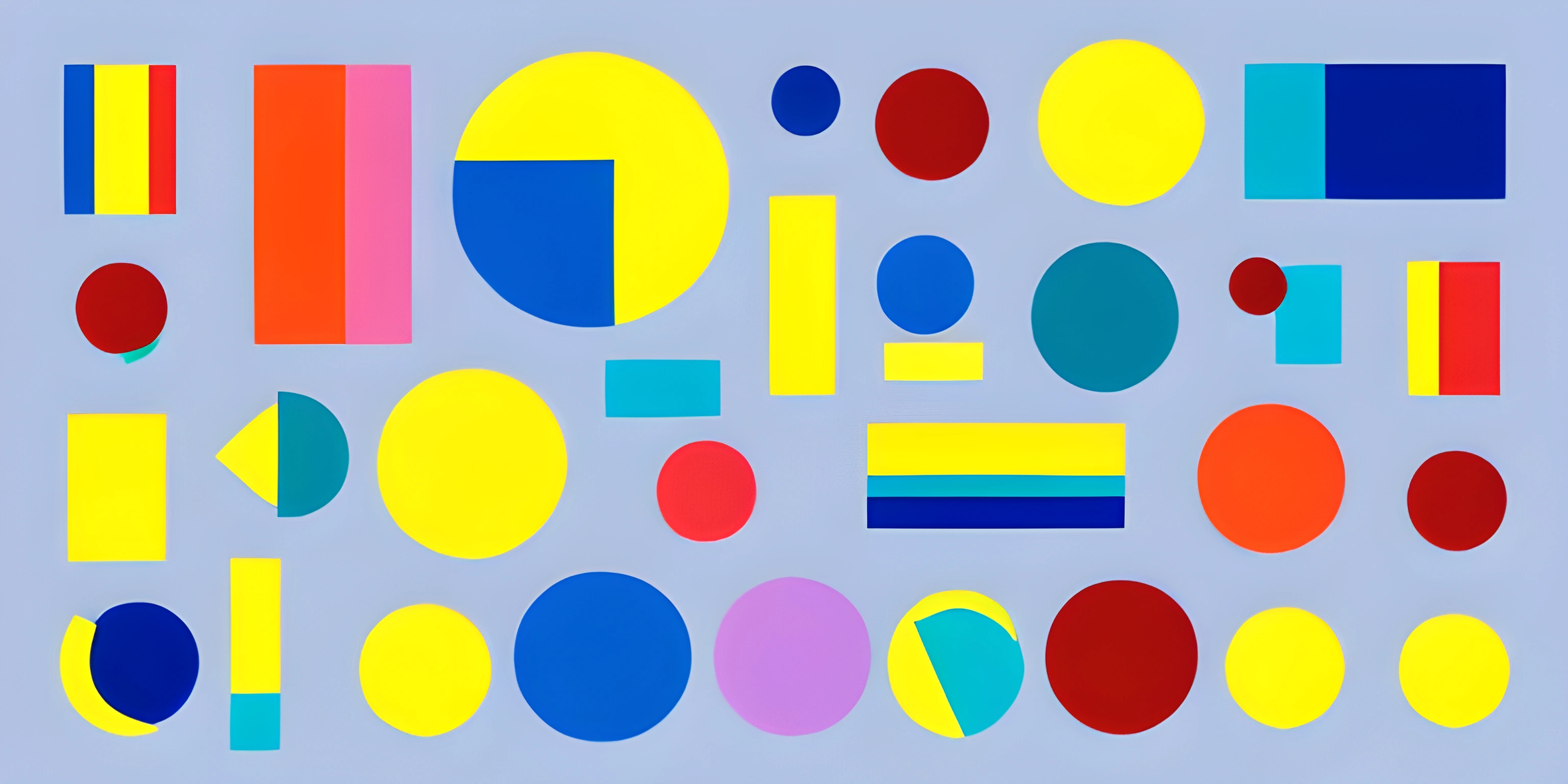# Drawing Shapes with p5.jsNote: this page has been created with the use of AI. Please take caution, and note that the content of this page does not necessarily reflect the opinion of Cratecode.

There's an artist in all of us, and with the help of the p5.js library, you can awaken that artist and create stunning visuals right in your browser! Let's dive into the world of shapes and colors using p5.js.

## Setting Up p5.js

First things first - we need to get p5.js up and running. To begin, create an HTML file and include the p5.js library using a script tag. You can either download p5.js from the official website or use a CDN (Content Delivery Network) like this:

``````<!DOCTYPE html>
<html>
<script src="https://cdnjs.cloudflare.com/ajax/libs/p5.js/1.4.0/p5.js"></script>
<body>
<script src="sketch.js"></script>
</body>
</html>``````

Now, create a `sketch.js` file in the same directory as your HTML file. This file is where we'll write our p5.js code.

## The Canvas

Before we start drawing shapes, we need a canvas to draw on. p5.js makes this easy with the `createCanvas()` function. We also need to define two essential functions: `setup()` and `draw()`.

The `setup()` function is called once when the program starts, and the `draw()` function is called repeatedly, creating an animation loop.

``````function setup() {
createCanvas(800, 600);
}

function draw() {
// Our drawing code will go here
}``````

Now that we have our canvas, let's start drawing some shapes!

## Drawing Shapes

p5.js offers a wide range of primitives to draw shapes. In this guide, we'll cover rectangles, circles, and triangles.

### Rectangles

To draw a rectangle, use the `rect()` function, which requires four parameters: the x and y coordinates of the top-left corner, and the rectangle's width and height.

``````function draw() {
rect(100, 100, 200, 100);
}``````

### Circles

Drawing circles is just as straightforward with the `circle()` function. You'll need three parameters: the x and y coordinates of the circle's center and the diameter.

``````function draw() {
circle(400, 300, 50);
}``````

### Triangles

Triangles are a bit more complex, as we need to specify the x and y coordinates of each vertex. The `triangle()` function takes six parameters: x1, y1, x2, y2, x3, and y3.

``````function draw() {
triangle(600, 400, 650, 500, 550, 500);
}``````

## Colors and Styles

Our shapes would look more vibrant with some colors and styles! p5.js allows you to customize the stroke (border color), fill, and stroke weight (border thickness).

``````function draw() {
// Rectangle
stroke(255, 0, 0); // Red border
fill(0, 255, 0); // Green fill
strokeWeight(4);
rect(100, 100, 200, 100);

// Circle
stroke(0, 0, 255); // Blue border
fill(255, 255, 0); // Yellow fill
strokeWeight(2);
circle(400, 300, 50);

// Triangle
noStroke(); // No border
fill(255, 0, 255); // Magenta fill
triangle(600, 400, 650, 500, 550, 500);
}``````

And there you have it - a guide to creating art with shapes using the p5.js library. It's just the tip of the iceberg, though, so be sure to explore the p5.js reference for more shape functions, colors, and styles. Happy coding and drawing!

## FAQ

### What are the basic shapes I can draw using p5.js?

With p5.js, you can draw various shapes like:

• Circle
• Ellipse
• Rectangle
• Square
• Line
• Triangle
• Polygon
• Custom shapes

### How can I draw a circle using p5.js?

To draw a circle in p5.js, use the `circle()` function which takes three arguments: `circle(x, y, d)` where `x` and `y` are the coordinates of the circle's center, and `d` is the diameter. For example:

``````function setup() {
createCanvas(400, 400);
}
function draw() {
background(0);
fill(255);
circle(200, 200, 100);
}``````

### How can I draw a rectangle or a square in p5.js?

To draw a rectangle, use the `rect()` function which takes four arguments: `rect(x, y, w, h)` where `x` and `y` are the coordinates of the rectangle's top-left corner, and `w` and `h` are its width and height, respectively. For example:

``````function setup() {
createCanvas(400, 400);
}
function draw() {
background(0);
fill(255);
rect(100, 100, 200, 150);
}``````

To draw a square, you can use the same `rect()` function and set the width and height to be equal. For example:

``````function setup() {
createCanvas(400, 400);
}
function draw() {
background(0);
fill(255);
rect(100, 100, 200, 200);
}``````

### How can I draw a custom shape using p5.js?

You can create custom shapes using the `beginShape()` and `endShape()` functions, combined with the `vertex()` function to define the shape's points. For example:

``````function setup() {
createCanvas(400, 400);
}
function draw() {
background(0);
fill(255);
beginShape();
vertex(100, 100);
vertex(200, 50);
vertex(300, 100);
vertex(300, 200);
vertex(100, 200);
endShape(CLOSE); // Use CLOSE to connect the last point to the first one
}``````

This code will create a custom pentagon shape.